Courses

SRMJEEE Maths Mock Test - 7

35 Questions MCQ Test SRMJEEE Subject Wise & Full Length Mock Tests | SRMJEEE Maths Mock Test - 7

Description
Attempt SRMJEEE Maths Mock Test - 7 | 35 questions in 50 minutes | Mock test for JEE preparation | Free important questions MCQ to study SRMJEEE Subject Wise & Full Length Mock Tests for JEE Exam | Download free PDF with solutions
QUESTION: 1

Solution:
QUESTION: 2

Solution:
QUESTION: 3

The value of f(0) so that f (x) = sinx / x is continuous at x = 0 is

Solution:
QUESTION: 4

The centroid of a triangle formed by the points (0, 0), (cosθ, sinθ) and (sinθ - cosθ) lies on th line y = 2x. Then, θ is

Solution:
QUESTION: 5

The term independent of x in the expansion of [x2-(1/3x)]9 is

Solution:
QUESTION: 6

The pole of the line 9x+y-28=0 with respect to the circle 2x2+2y2-3x+5y-7=0 is

Solution:
QUESTION: 7

At which point of the parabola y2=4x is a normal drawn so that it makes equal angles with the axes?

Solution:
QUESTION: 8

If z = -2/(1 + √3i),then the value of arg (z) is

Solution:
QUESTION: 9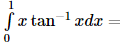Solution:
QUESTION: 10

A singular solution of the differential equation y2[1+(dy/dx)2]=R2 is :

Solution:
QUESTION: 11

(d/dx)(log tanx)=

Solution:
QUESTION: 12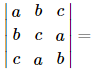Solution:
QUESTION: 13

cos(sin⁻13/5)+sin⁻1(5/13))=

Solution:
QUESTION: 14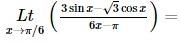Solution:
QUESTION: 15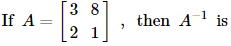Solution:
QUESTION: 16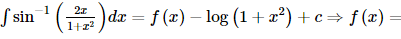Solution:
QUESTION: 17

The inverse of a matrix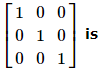Solution:
QUESTION: 18

The maximum value of x3-3x in the interval [0,2] is

Solution:
QUESTION: 19

Mean of a set of values is based on:

Solution:
QUESTION: 20

One series containing 3 numbers has mean 8, variance 24 and the second series containing 5 numbers has mean 8, variance 18. Then the variance of the combined data is

Solution:
QUESTION: 21

If angle between the lines 2x2+5xy+3y2+6x+7y+4=0 is tan⁻1m, the value of m is

Solution:
QUESTION: 22

In how many different ways can the letters of the word RUMOUR be arranged?

Solution:
QUESTION: 23

What is the chance of getting multiple of 2 on one and multiple of 3 on the other in a single throw of two dice?

Solution:
QUESTION: 24

In a Δ ABC , A : B : C = 3 : 5 : 4 . Then a + b + c√2 is equal to

Solution: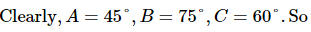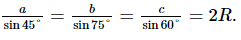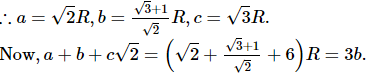QUESTION: 25

The value of λ for which one root of 3x2 - (1 + 4λ)x + λ2 + 2 = 0 may be one-third of the other is

Solution:
QUESTION: 26

In Rule Method the null set is represented by

Solution:
QUESTION: 27

If p, q, r are in A.P. and x, y, z are in G.P., then xq-r yr-p zp-q is equal to

Solution:

p, q, r are in A.P. Let d be the c.d.
∴ q = p + d and r = p + 2d
Also, x, y, z are in G.P. Let R be the c.r.
∴ y = xR and z = xR2
Putting the values of q, r, y, z in xq-r yr-p zp-q, we get
= xq-r yr-p zp-q = x(p + d) - (p + 2d) (xR)(p + 2d) - p (xR2)p - (p + d)
= x-d (xR)2d (xR2)-d = x-d. x2dR2d . x-dR-2d
= x-d + 2d - d . R2d - 2d = x0R0 = 1 x 1 = 1

QUESTION: 28

The derivative of sin2 x with respect to (log x)2 is

Solution:
QUESTION: 29

The equation of bisectors between the lines 3x+4y-7=0 and 12x+5y+17=0 are

Solution:
QUESTION: 30

The equation of the tangent to the curve y=2 sin x+sin 2x at x=π/3 is equal to

Solution:
QUESTION: 31

The equation sinx + siny + sinz = -3 for 0≤x≤2π, 0≤y≤2π, 0≤z≤2π, has

Solution:
QUESTION: 32

If A lies in the 2nd quadrant and 3 tan A+4=0 then the value of 2 cot A-5 cos A+sinA is equal to

Solution:
QUESTION: 33

A variable has Poisson distribution with mean m. The probability that the variable takes any of the values 0 or 2 is

Solution:
QUESTION: 34

If a=i+2j-2k, b=2i-j+k, c=i+3j-k, then ax(bxc)=

Solution:
QUESTION: 35

If vectors i-3j+2k, -i+2j represent the diagonals of a parallelogram, then its area is

Solution:Use Code STAYHOME200 and get INR 200 additional OFF Use Coupon Code

Track your progress, build streaks, highlight & save important lessons and more!

Similar ContentRelated tests